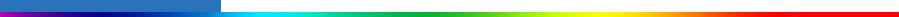Working Group I: The Scientific BasisGet Javascript Other reports in this collection

#### 8.5.1.2.1 Surface quantities

The SAR’s evaluation of coupled-model simulations focused on surface air temperature, sea level pressure and precipitation. The SAR concluded that model simulations of surface air temperature were “very similar” to observations. Simulations of the other two quantities were found to be less accurate but nevertheless reasonable: the SAR concluded that coupled models represented “the observed large-scale geographical distribution” of sea level pressure “rather well”, and that they were “generally successful in simulating the broad-scale structure of the observed precipitation”.Figure 8.2: December-January-February climatological surface air temperature in K simulated by the CMIP1 model control runs. Averages over all models (upper left), over all flux adjusted models (lower left) and over all non-flux adjusted models (lower right) are shown together with zonal mean values for individual models (upper right). Observed value is shown in the zonal mean plot (thick solid line), and the difference between “average model” and observation is shown on the longitude-latitude maps. From Lambert and Boer (2001).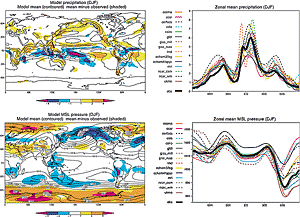Figure 8.3: December-January-February climatological precipitation in mm per day (top) and mean sea level pressure in hPa (bottom) simulated by CMIP1 model control runs. Averages over all models are shown at left and zonal mean values for individual models are shown at right. Observed values are shown on the zonal mean plot (thick solid line) and the difference between an “average model” and the observations is shown by shading on the longitude-latitude maps. From Lambert and Boer (2001).

Figures 8.2 and 8.3 (reproduced from Lambert and Boer, 2001) update this assessment using coupled model output from the CMIP1 database. For each quantity, the figures show both a map of the average over all fifteen models (“model mean”) and zonal means for all individual fifteen models. Lambert and Boer (2001) demonstrate that the model mean exhibits good agreement with observations, often better than any of the individual models. Inspection of these portions of the figures reaffirms the SAR conclusions summarised above. The errors in model-mean surface air temperature rarely exceed 1°C over the oceans and 5°C over the continents; precipitation and sea level pressure errors are relatively greater but the magnitudes and patterns of these quantities are recognisably similar to observations. The bottom portion of Figure 8.2 shows maps of the model mean taken separately over all flux adjusted models (lower left) and all non-flux adjusted models (lower right). Flux adjusted models are generally more similar to the observations - and to each other - than are non-flux adjusted models. However, errors in the non-flux adjusted model mean are not grossly larger than errors in the flux adjusted model mean (except in polar regions). This result from the “inter-model” CMIP database suggests that the SAR was correct in anticipating that the need for flux adjustments would diminish as coupled models improve. It is reinforced by “intra-model ensembles”, i.e., by the experience that improvements to individual models can reduce the need for flux adjustments (e.g., Boville and Gent, 1998).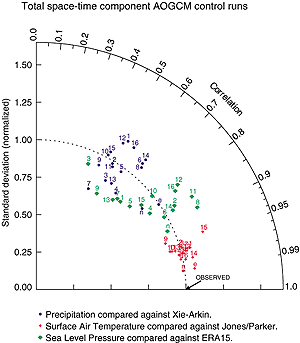Figure 8.4: Second-order statistics of surface air temperature, sea level pressure and precipitation simulated by CMIP2 model control runs. The radial co-ordinate gives the magnitude of total standard deviation, normalised by the observed value, and the angular co-ordinate gives the correlation with observations. It follows that the distance between the OBSERVED point and any model’s point is proportional to the r.m.s model error (see Section 8.2). Numbers indicate models counting from left to right in the following two figures. Letters indicate alternate observationally based data sets compared with the baseline observations: e = 15-year ECMWF reanalysis (“ERA”); n = NCAR/NCEP reanalysis. From Covey et al. (2000b).

The foregoing points are made in a more quantitative fashion by Figures 8.4 to 8.6 (reproduced from Covey et al., 2000b). Figure 8.4 gives the standard deviation and correlation with observations of the total spatial and temporal variability (including the seasonal cycle, but omitting the global mean) for surface air temperature, sea level pressure and precipitation in the CMIP2 simulations. The standard deviation is normalised to its observed value and the correlation ranges from zero along an upward vertical line to unity along a line pointing to the right. Consequently, the observed behaviour of the climate is represented by a point on the horizontal axis a unit distance from the origin. In this coordinate system, the linear distance between each model’s point and the “observed” point is proportional to the r.m.s. model error (Taylor, 2000; see also Box 8.1). Surface air temperature is particularly well simulated, with nearly all models closely matching the observed magnitude of variance and exhibiting a correlation > 0.95 with the observations. Sea level pressure and precipitation are simulated less well, but the simulated variance is still within ±25% of observed and the correlation with observations is noticeably positive (about 0.7 to 0.8 for sea level pressure and 0.4 to 0.6 for precipitation).

Observational uncertainties are indicated in Figure 8.4 by including extra observational data sets as additional points, as if they were models. These additional points exhibit greater agreement with the baseline observations as expected. It is noteworthy, however, that the differences between alternate sets of observations are not much smaller than the differences between models and the baseline observations. This result implies that in terms of variance and space-time pattern correlation, the models nearly agree with observations to within the observational uncertainty.

Figures 8.5 and 8.6 show global mean errors (“bias”) and root mean square (r.m.s.) errors normalised by standard deviations for surface air temperature and precipitation in CMIP2 model simulations. Both the r.m.s. errors and the background standard deviations are calculated from the full spatial and temporal variability of the fields. The r.m.s. errors are divided into a number of components such as zonal mean vs. deviations and annual mean vs. seasonal cycle. For nearly all models the r.m.s. error in zonal- and annual-mean surface air temperature is small compared with its natural variability. The errors in the other components of surface air temperature, and in zonal mean precipitation, are relatively larger but generally not excessive compared with natural variability.

In Figures 8.5 and 8.6, models are divided into flux adjusted and non-flux adjusted classes. Slight differences between the two may be discerned, but it is not obvious that these are statistically significant if the two classes are considered as random samples from a large population of potential climate models. The same conclusion was reached in a detailed study of the seasonal cycle of surface air temperature in the CMIP models (Covey et al., 2000a). (That study, however, also noted that many of the non-flux adjusted models suffered from unrealistic “climate drift” up to about 1°C / century in global mean surface temperature.) The relatively small differences between flux adjusted and non-flux adjusted models noted above suggest that flux adjustments could be - indeed, are being - dispensed with at acceptable cost in many climate models, at least for the century time-scale integrations of interest in detecting and predicting anthropogenic climate change. In recent models that omit flux adjustment, the representation of atmospheric fields has in some cases actually improved, compared with older, flux-adjusted versions of the models. Examples include the HadCM3 model and the CSM 1.0 model. In CSM 1.0, atmospheric temperature, precipitation and atmospheric circulation are close to values simulated when the atmospheric component of the CSM 1.0 model is driven by observed sea surface temperatures (Boville and Hurrell, 1998).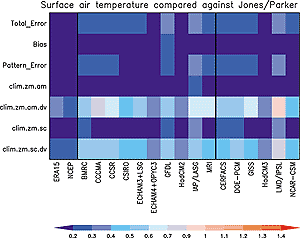Figure 8.5: Components of space-time errors of surface air temperature (climatological annual cycle) simulated by CMIP2 model control runs. Shown are the total errors, the global and annual mean error (“bias”), the total r.m.s (“pattern”) error, and the following components of the climatological r.m.s. error: zonal and annual mean (“clim.zm.am”); annual mean deviations from the zonal mean (“clim.zm.am.dv”), seasonal cycle of the zonal mean (“clim.zm.sc”); and seasonal cycle of deviations from the zonal mean (“clim.zm.sc.dv”). For each component, errors are normalised by the component’s observed standard deviation. The two left-most columns represent alternate observationally based data sets, ECMWF and NCAR/NCEP reanalyses, compared with the baseline observations (Jones et al., 1999). Remaining columns give model results: the ten models to the left of the second thick vertical line are flux adjusted and the six models to the right are not. From Covey et al. (2000b).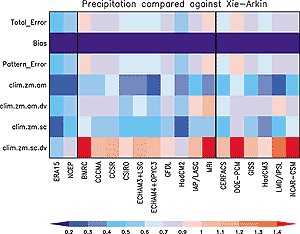Figure 8.6: As in the Figure 8.5, for precipitation. The two left-most columns represent alternate observationally based data sets, 15-year ECMWF reanalysis (“ECMWF”) and NCAR/NCEP reanalysis (“NCEP”), compared with the baseline observations (Xie and Arkin, 1996). From Covey et al. (2000b).

 Box 8.1: Taylor diagrams To quantify how well models simulate an observed climate field, it is useful to rely on three non-dimensional statistics: the ratio of the variances of the two fields:2 =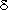2mod/2obs the correlation between the two fields (R, which is computed after removing the overall means), and the r.m.s difference between the two fields (E, which is normalised by the standard deviation of the observed field). The ratio of variance indicates the relative amplitude of the simulated and observed variations, whereas the correlation indicates whether the fields have similar patterns of variation, regardless of amplitude. The normalised r.m.s error can be resolved into a part due to differences in the overall means (E0), and a part due to errors in the pattern of variations (E'). These statistics provide complementary, but not completely independent, information. Often the overall differences in means (E0) is reported separately from the three pattern statistics (E',, and R), but they are in fact related by the following equation: E'2 = E2 - E02 = 1 +2-2R This relationship makes it possible to display the three pattern statistics on a two-dimensional plot like that in Figure 8.4. The plot is constructed based on the Law of Cosines. The observed field is represented by a point at unit distance from the origin along the abscissa. All other points, which represent simulated fields, are positioned such thatis the radial distance from the origin, R is the cosine of the azimuthal angle, and E' is the distance to the observed point. When the distance to the point representing the observed field is relatively short, good agreement is found between the simulated and observed fields. In the limit of perfect agreement (which is, however, generally not achievable because there are fundamental limits to the predictability of climate), E' would approach zero, andand R would approach unity.